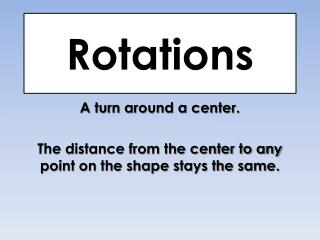# Rotations - PowerPoint PPT PresentationDownload PresentationRotations

RotationsDownload Presentation## Rotations

- - - - - - - - - - - - - - - - - - - - - - - - - - - E N D - - - - - - - - - - - - - - - - - - - - - - - - - - -
##### Presentation Transcript

1. Rotations A turn around a center. The distance from the center to any point on the shape stays the same.

2. Rotations  degrees & direction Clockwise

3. A rotation turns a figure through an angle about a fixed point called the center.  It is a rigid isometry. Rules of rotation are for clockwise rotations. Counter clockwise rotations are opposite clockwise. 90°cw = 270°ccw and 270°cw = 90°ccw

4. Rotate ∆TSN 90°cw (x, y)  (y, -x) T’ T(-1, 1)  T'(1, 1) S(4, -1)  S'(-1, -4) N(1, -4)  N'(-4, -1) N’ S’ (270 ° CCW rotation)

5. Rotate ∆TSN 180° (x, y) (-x, -y) T(-1, 1)  T'(1, -1)S(4, -1)  S'(-4, 1)N(1, -4)  N'(-1, 4)

6. Rotate ∆TSN270° cw (x, y) to (-y, x) T(-1, 1) T'(-1, -1)S(4, -1) S'(1, 4)N(1, -4) N'(4, 1)

7. Rotate 90 CW about the Origin(Same as 270 CCW) Change the sign of x and switch the order

8. Rotate 90 CW

9. Rotate 270Clockwise(Same as 90ccw) Change the sign of y and switch the order

10. Rotate 90° counterclockwiseabout the origin

11. Rotate 90° counterclockwiseabout the origin

12. Rotate 180about the Origin ONLY Change the signs

13. Rotate 180° about the origin

14. Rotate 180° about the origin

15. A rotation turns a figure through an angle about a fixed point called the center.  It is a rigid isometry. Rules of rotation are for clockwise rotations. Counter clockwise rotations are opposite clockwise. 90°cw = 270°ccw and 270°cw = 90°ccw

16. Virtual Nerd Tutoring Lessons Lesson on Rotations http://www.virtualnerd.com/pre-algebra/geometry/transformations-symmetry/define-transformations/rotation-definition Lesson on Rotations 90° http://www.virtualnerd.com/pre-algebra/geometry/transformations-symmetry/rotating-figures/rotate-90-degrees-about-origin Lesson on Rotations 180° http://www.virtualnerd.com/pre-algebra/geometry/transformations-symmetry/rotating-figures/rotate-180-degrees-about-origin

17. Coordinate Rules for Rotations about the origin: When a point (x, y) is rotated clockwise about the origin, the following rules are true: • For a rotation of 900(x, y)  (y, -x). • For a rotation of 1800(x,y)  (-x, -y). • For a rotation of 2700(x,y)  (-y, x). • When a point (x, y) is rotated counterclockwise about the origin, the following rules are true: • For a rotation of 900 (x,y) (-y, x). • For a rotation of 1800(x,y) (-x, -y). • For a rotation of 2700 (x, y) (y, -x).